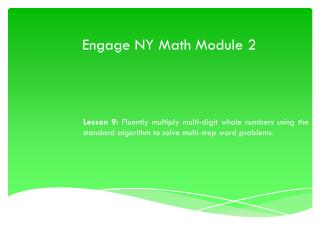Download PresentationEngage NY Math Module 2

# Engage NY Math Module 2 - PowerPoint PPT Presentation

Engage NY Math Module 2. Lesson 9: Fluently multiply multi-digit whole numbers using the standard algorithm to solve multi-step word problems. . Multiply and Divide by Exponents. Write 45 tenths as a decimal. 4.5 Multiply by 4.5 x = 450 Calculate the following: 0.4 x 0.4 ÷I am the owner, or an agent authorized to act on behalf of the owner, of the copyrighted work described.
Download Presentation## Engage NY Math Module 2

An Image/Link below is provided (as is) to download presentation

Download Policy: Content on the Website is provided to you AS IS for your information and personal use and may not be sold / licensed / shared on other websites without getting consent from its author.While downloading, if for some reason you are not able to download a presentation, the publisher may have deleted the file from their server.

- - - - - - - - - - - - - - - - - - - - - - - - - - E N D - - - - - - - - - - - - - - - - - - - - - - - - - -
Presentation Transcript
1. Engage NY Math Module 2 Lesson 9: Fluently multiply multi-digit whole numbers using the standard algorithm to solve multi-step word problems.

2. Multiply and Divide by Exponents • Write 45 tenths as a decimal. • 4.5 • Multiply by • 4.5 x = 450 • Calculate the following: • 0.4 x • 0.4 ÷ • 3.895 x • 3.895 ÷

3. Estimate the Product • 421 x 231 ≈ ____ x ____ = ____ • Round both factors to the nearest hundred. • 421 x 231 ≈ 400 x 200= ____ • What’s 400 x 200? • 80,000 • 523 x 298 ≈ ____ x ____ = ____ • Round both factors to the nearest hundred. • 523 x 298 ≈ 500x 300= ____ • What’s 500 x 300? • 150,000

4. Estimate the Product • 684 x 347 ≈ ____ x ____ = ____ • Round both factors to the nearest hundred. • 684 x 347 ≈ 700x 300= ____ • What’s 700 x 300? • 210,000 • 908 x 297 ≈ ____ x ____ = ____ • Round both factors to the nearest hundred. • 908 x 297 ≈ 900x 300= ____ • What’s 900 x 300? • 270,000

5. Concept Development – Problem 1: • An office space in New York City measures 48 feet by 56 feet. If it sells for \$565 per square foot, what is the selling price of the office space? • We will work Problem 1 together. Let’s read the word problem aloud. • Now let’s reread the problem sentence by sentence and draw as we go. • “An office space in New York City measures 48 feet by 56 feet.” • What do you see? Can we draw something? • Read the next sentence. • “If it sells for \$565 per square foot, what is the selling price of the office space?” • What is the important information and how can we show this in our drawing? • The office square sells for \$565 for each square foot. We can draw a single square unit inside our rectangle to remind us. • We can write that 1 unit = \$565 48 ft. 56 ft.

6. Concept Development – Problem 1: • An office space in New York City measures 48 feet by 56 feet. If it sells for \$565 per square foot, what is the selling price of the office space? • How do we solve this problem? Turn and talk. • We have to multiply. • We have to find the total square feet of the office space then multiply by \$565. • We have to first find the area of the office space then multiply by \$565. • What information are we given that would help us figure out the area? • We can multiply the length times the width. • Find the area of the office space. • A = LxW • A = 56 x 48 • A = 2,688 ft² • Have we answered the question? 48 ft. 56 ft. 1 unit = \$565

7. Concept Development – Problem 1: • An office space in New York City measures 48 feet by 56 feet. If it sells for \$565 per square foot, what is the selling price of the office space? • A = 2,688 ft² • Have we answered the question? • No. We need to multiply the area by the cost of one square foot, \$565, to find the total cost. • Solve and express your answer using a statement of solution. 48 ft. 56 ft. 2, 6 8 8 X 5 6 5 1 3, 4 4 0 1 6 1, 2 8 0 +1, 3 4 4, 0 0 0 1, 5 1 8, 7 2 0 1 unit = \$565 2,688 units = 2,688 x 565 The selling price of the office space was \$1,518,720.

8. Concept Development – Problem 2: • Gemma and Leah are both jewelry makers. Gemma made 106 beaded necklaces. Leah made 39 more necklaces than Gemma. • Each necklace they make has exactly 104 beads on it. How many beads did both girls use while making their necklaces? • At a recent craft fair, Gemma sold her necklaces for \$14 each. Leah sold her necklaces for \$10 more. Who made more money at the craft fair? How much more? • Reread the problem sentence by sentence. • What do you see? Can we draw something? • Yes. • What can we draw? • A bar for Gemma’s necklaces and a second, longer bar for Leah’s. • Draw and label your tape diagrams.